# RS Aggarwal Solutions for Class 7 Maths Exercise 20C Chapter 20 Mensuration

The RS Aggarwal Solutions for Class 7 Maths Exercise 20C Chapter 20 Mensuration, contains different questions with various solutions to the problems. This exercise of RS Aggarwal Solutions for Class 7 Chapter 20 includes questions related to the area of a parallelogram and the area of rhombus. Students have to practice RS Aggarwal Solutions for Class 7 Chapter 20 regularly and become an expert in the Maths. To strengthen the fundamentals and be able to solve RS Aggarwal Solutions for Class 7 Maths Chapter 20 Mensuration questions that are usually asked in the final exam.

## Download the PDF of RS Aggarwal Solutions For Class 7 Maths Chapter 20 Mensuration – Exercise 20C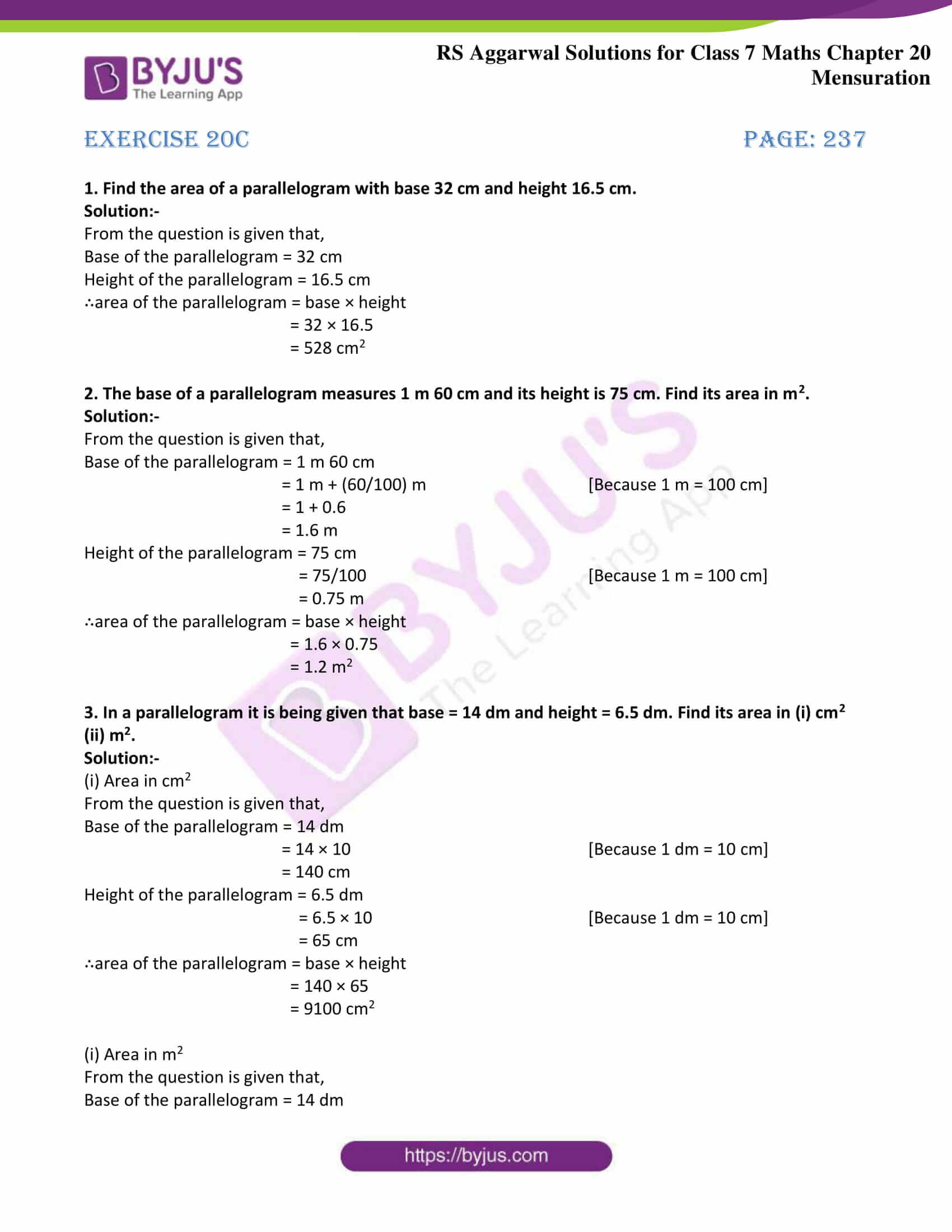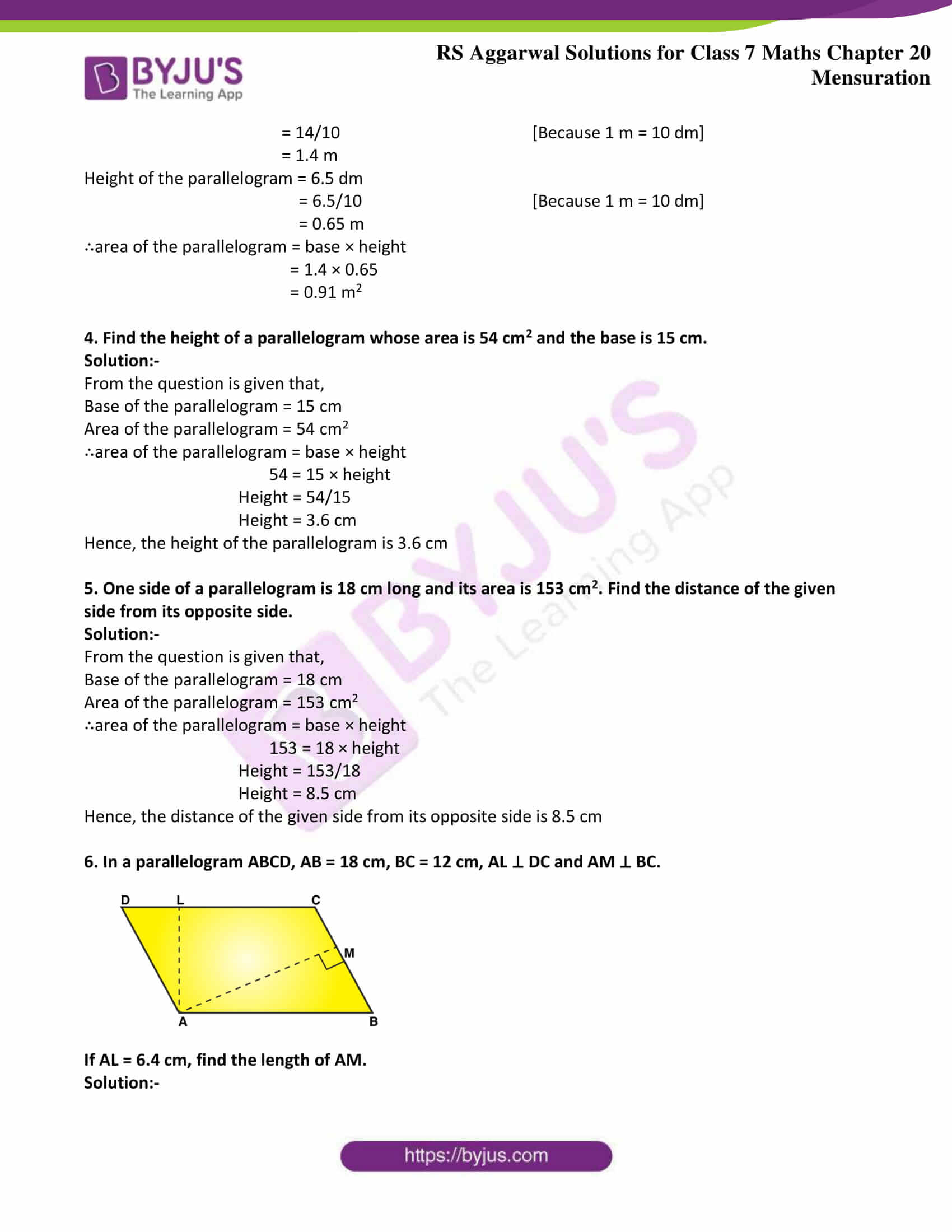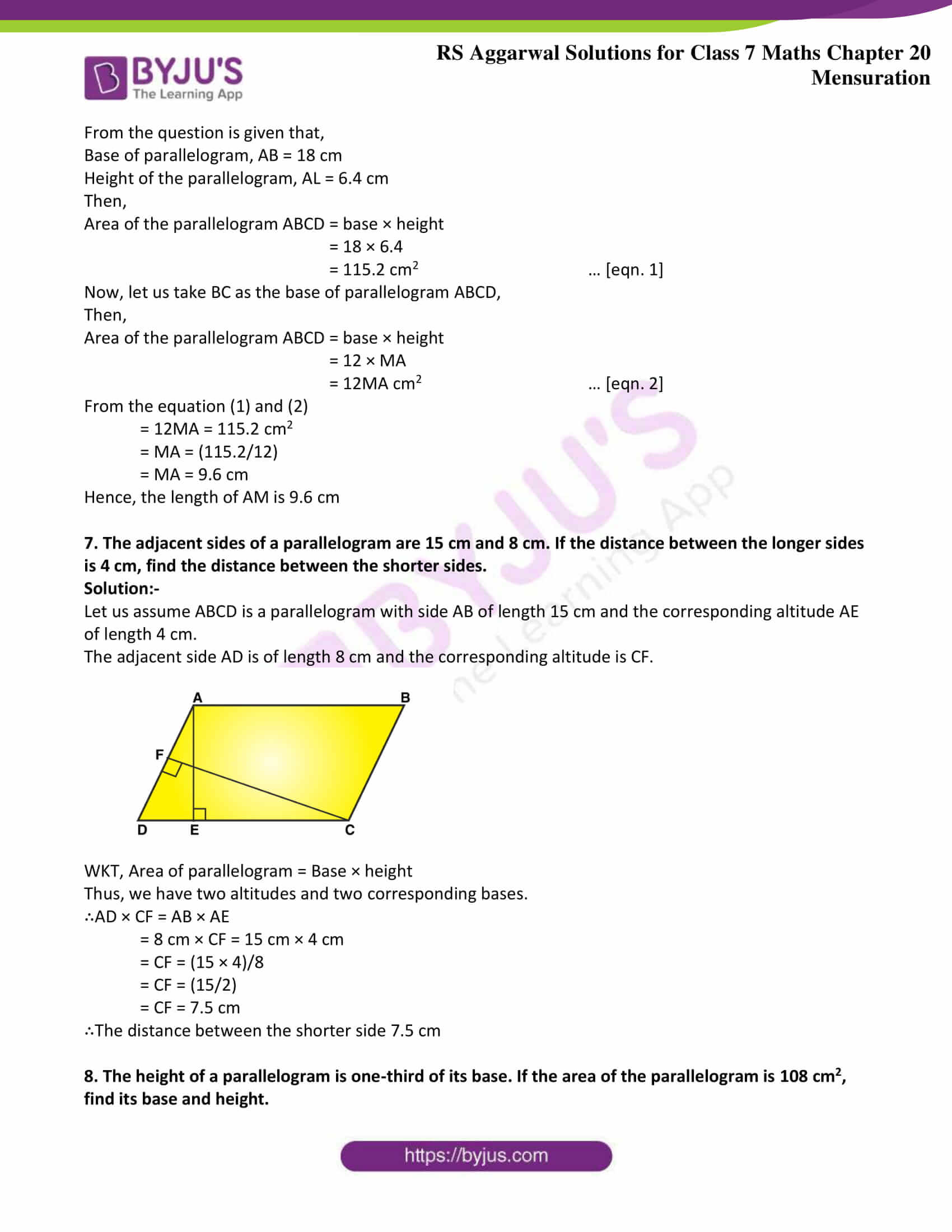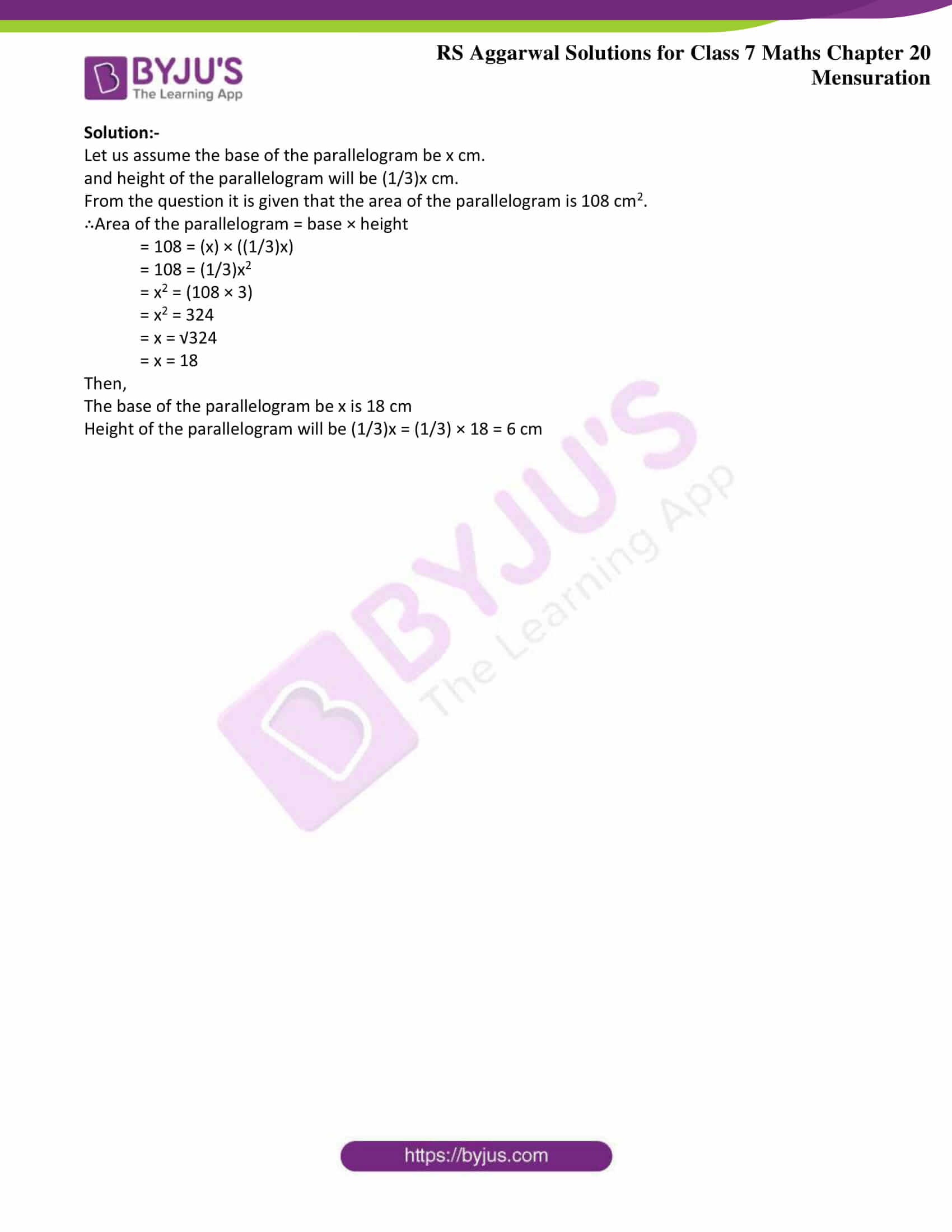### Access answers to Maths RS Aggarwal Solutions for Class 7 Chapter 20 – Mensuration Exercise 20C

1. Find the area of a parallelogram with base 32 cm and height 16.5 cm.

Solution:-

From the question is given that,

Base of the parallelogram = 32 cm

Height of the parallelogram = 16.5 cm

∴area of the parallelogram = base × height

= 32 × 16.5

= 528 cm2

2. The base of a parallelogram measures 1 m 60 cm and its height is 75 cm. Find its area in m2.

Solution:-

From the question is given that,

Base of the parallelogram = 1 m 60 cm

= 1 m + (60/100) m [Because 1 m = 100 cm]

= 1 + 0.6

= 1.6 m

Height of the parallelogram = 75 cm

= 75/100 [Because 1 m = 100 cm]

= 0.75 m

∴area of the parallelogram = base × height

= 1.6 × 0.75

= 1.2 m2

3. In a parallelogram it is being given that base = 14 dm and height = 6.5 dm. Find its area in (i) cm2

(ii) m2.

Solution:-

(i) Area in cm2

From the question is given that,

Base of the parallelogram = 14 dm

= 14 × 10 [Because 1 dm = 10 cm]

= 140 cm

Height of the parallelogram = 6.5 dm

= 6.5 × 10 [Because 1 dm = 10 cm]

= 65 cm

∴area of the parallelogram = base × height

= 140 × 65

= 9100 cm2

(i) Area in m2

From the question is given that,

Base of the parallelogram = 14 dm

= 14/10 [Because 1 m = 10 dm]

= 1.4 m

Height of the parallelogram = 6.5 dm

= 6.5/10 [Because 1 m = 10 dm]

= 0.65 m

∴area of the parallelogram = base × height

= 1.4 × 0.65

= 0.91 m2

4. Find the height of a parallelogram whose area is 54 cm2 and the base is 15 cm.

Solution:-

From the question is given that,

Base of the parallelogram = 15 cm

Area of the parallelogram = 54 cm2

∴area of the parallelogram = base × height

54 = 15 × height

Height = 54/15

Height = 3.6 cm

Hence, the height of the parallelogram is 3.6 cm

5. One side of a parallelogram is 18 cm long and its area is 153 cm2. Find the distance of the given side from its opposite side.

Solution:-

From the question is given that,

Base of the parallelogram = 18 cm

Area of the parallelogram = 153 cm2

∴area of the parallelogram = base × height

153 = 18 × height

Height = 153/18

Height = 8.5 cm

Hence, the distance of the given side from its opposite side is 8.5 cm

6. In a parallelogram ABCD, AB = 18 cm, BC = 12 cm, AL ⊥ DC and AM ⊥ BC.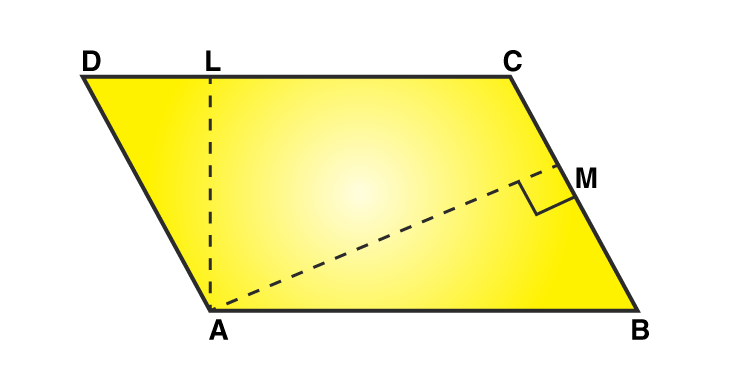If AL = 6.4 cm, find the length of AM.

Solution:-

From the question is given that,

Base of parallelogram, AB = 18 cm

Height of the parallelogram, AL = 6.4 cm

Then,

Area of the parallelogram ABCD = base × height

= 18 × 6.4

= 115.2 cm2 … [eqn. 1]

Now, let us take BC as the base of parallelogram ABCD,

Then,

Area of the parallelogram ABCD = base × height

= 12 × MA

= 12MA cm2 … [eqn. 2]

From the equation (1) and (2)

= 12MA = 115.2 cm2

= MA = (115.2/12)

= MA = 9.6 cm

Hence, the length of AM is 9.6 cm

7. The adjacent sides of a parallelogram are 15 cm and 8 cm. If the distance between the longer sides is 4 cm, find the distance between the shorter sides.

Solution:-

Let us assume ABCD is a parallelogram with side AB of length 15 cm and the corresponding altitude AE of length 4 cm.

The adjacent side AD is of length 8 cm and the corresponding altitude is CF.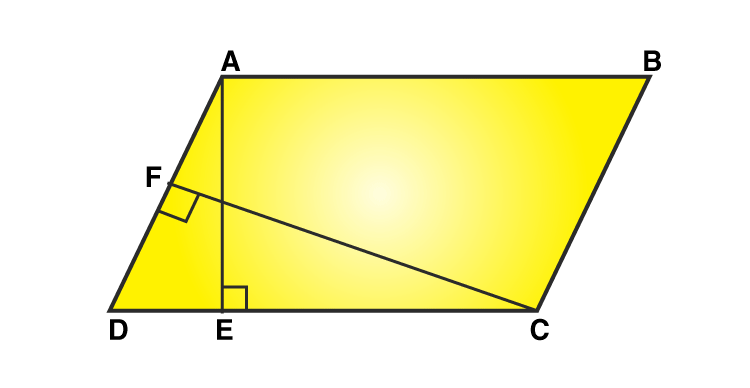WKT, Area of parallelogram = Base × height

Thus, we have two altitudes and two corresponding bases.

∴AD × CF = AB × AE

= 8 cm × CF = 15 cm × 4 cm

= CF = (15 × 4)/8

= CF = (15/2)

= CF = 7.5 cm

∴The distance between the shorter side 7.5 cm

8. The height of a parallelogram is one-third of its base. If the area of the parallelogram is 108 cm2, find its base and height.

Solution:-

Let us assume the base of the parallelogram be x cm.

and height of the parallelogram will be (1/3)x cm.

From the question it is given that the area of the parallelogram is 108 cm2.

∴Area of the parallelogram = base × height

= 108 = (x) × ((1/3)x)

= 108 = (1/3)x2

= x2 = (108 × 3)

= x2 = 324

= x = √324

= x = 18

Then,

The base of the parallelogram be x is 18 cm

Height of the parallelogram will be (1/3)x = (1/3) × 18 = 6 cm

### Access other exercises of RS Aggarwal Solutions For Class 7 Chapter 20 – Mensuration

Exercise 20A Solutions

Exercise 20B Solutions

Exercise 20D Solutions

Exercise 20E Solutions

Exercise 20F Solutions

Exercise 20G Solutions